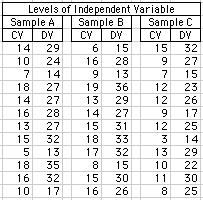Analysis of Covariance for 3 Independent Samples
ForImporting Data from a Spreadsheet

 The logic and computational details of the one-way independent-samples ANCOVA are described in Chapter 17 of Concepts and Applications.

This page will perform an analysis of covariance for three independent samples, A, B, and C, where
• A, B, and C represent three quantitative or categorical levels of the independent variable;
• DV = the dependent variable of interest; and
• CV = the concomitant variable whose effects one wishes to bring under statistical control.
Procedure: When importing data from a spreadsheet, the paired values of CV and DV for each sample must be in the form of two adjacent columns, as shown in the following figure, with the CV for each sample on the left and the DV on the right. Within the spreadsheet application, select and copy the two columns of data for Sample A.Then return to this page, click the cursor into the data-entry field for Sample A, below, and perform the 'Paste' operation. Do the same for the other two samples in your analysis, then click the 'Calculate' button.

For each sample, make sure that the final entry is not followed by an extra line. (An extra line after the final entry in a sample will be interpreted as an extra data entry whose value is zero.) After all values for a sample have been entered, click the cursor immediately to the right of the final entry in the list, then press the down-arrow key. If an extra line is present, the cursor will move downward. Extra lines can be removed by pressing the down arrow key until the cursor no longer moves, and then pressing the 'Backspace' key (on a Mac platform, 'delete') until the cursor stands immediately to the right of the final entry.

Data Entry:
CV = concomitant variable [left column in each sample]
DV = dependent variable [right column in each sample]
 Levels of Independent Variable Sample A Sample B Sample C

 Dependent Variable Sample Total A B C n Observed Means Adjusted Means

 Aggregate Correlation within Samples: CV vs DV r = r2 =

ANCOVA Summary